# Follow Bolted joint comparison to Eurocode - 2

Peter, I read, read, read about bolts, the bolt's enginerring stress. But the problem is the same, I could not see the plasticity occurring in the graph Moment X Rotation.

1 First point, I do not agree when the people specify tightening a fastener to its yield point. Because I see it as a problem, whcih tease the yiled point in the other components, as column and end plate, before that it shall occure.

2 Second point, comparing with eurocode, it is similar, but I would like to see the point which change the stiffness, or in the graph the inclination, the plot shall not be linear.

3 Third point, I think taht it occures because the bolt pretension is so high, but it is the minimun normative loads. So the yield shall not ocure.

4 Fourth and last point. I think that the problem is in the egineering stress in the bolts.

Is attached: the graph moment rotation results, the engineering stress table, the lecture which I took the engineering stress, and the model. And finnaly the mathcad that I did, to comparte the graph moment X rotation.

If anybody could help me, I would be greatful.

• edited August 2018

• I also don’t agree that tightening a fastener to its yield point is the best practice. I only said that some people do that.

If I suppress one symmetry BC, I can convert this problem from a beam supported by two columns to a cantilever beam supported from a single column. This represents the condition in the original paper that has the nonlinear plot.  Below is the plot of the pressure vs. deformation from your model with the suppressed symmetry BC. From previous posts, you know that a simple scale factor can convert deformation to rotation and another scale factor can convert pressure to moment.  This plot shows the nonlinear response you were looking for.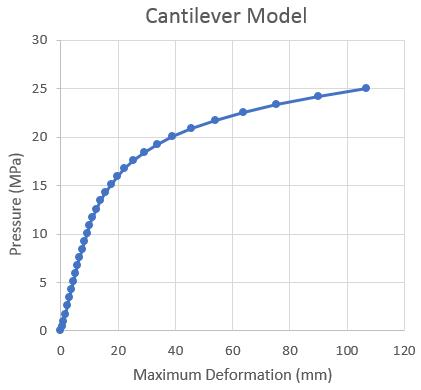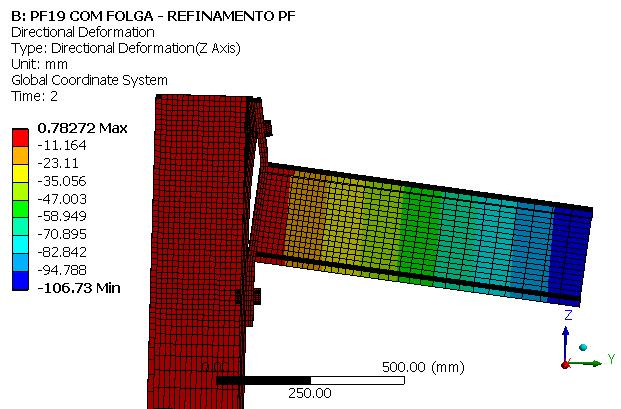Regards,

Peter

• Fabricio,

Note that in the bolt model, there is a distribution of stress over the volume and a stress singularity under the head at the perfectly sharp interior corner and at the nut that is bonded to the bolt. These stress singularities create a high local stress value that is much higher than the nominal stress through the shaft of the bolt.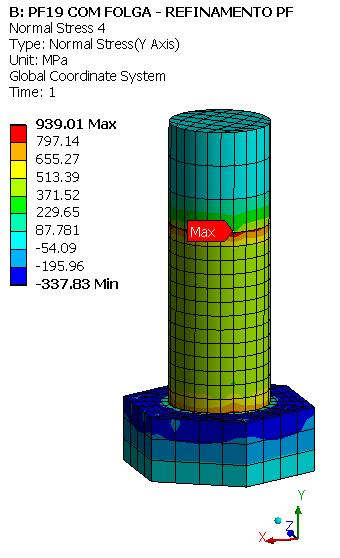The 939 MPa peak stress in the above plot is practically irrelevant.

In order to see the stress through the shaft of the bolt, away from the stress singularity at the head and nut, a Surface is used to plot the Normal Y stress.

The bolt shaft is split to apply the pretension, so we don't want the surface at the same location as the pretension split which is shown below.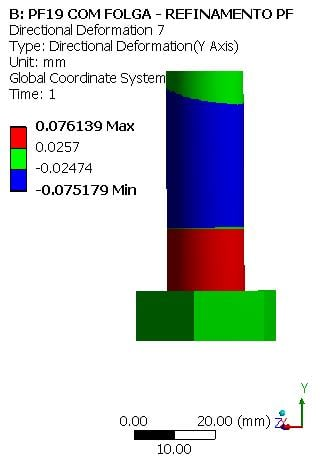The coordinate system shows where the surface is located, between the nut and the pretension split.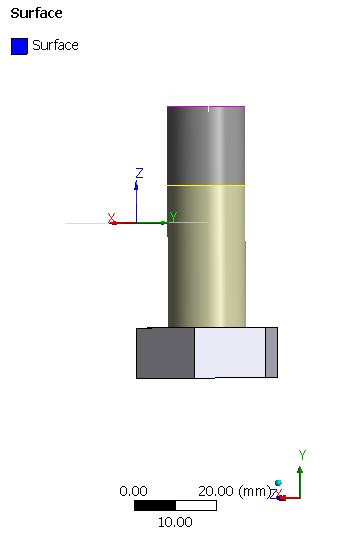Below is a plot of the stress on the elements passing through that surface. Note that the stress through the shaft of the bolt is in the 400 to 500 MPa range, with an average of 443 MPa, since the force is 125000 N and the area is 282 mm^2.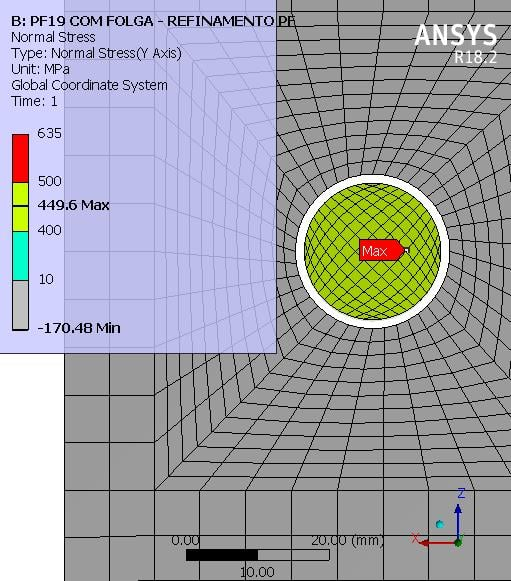The average stress is a lot lower than the yield strength of 635 MPa.  Below is the multilinear hardening plot for the bolt material, A325.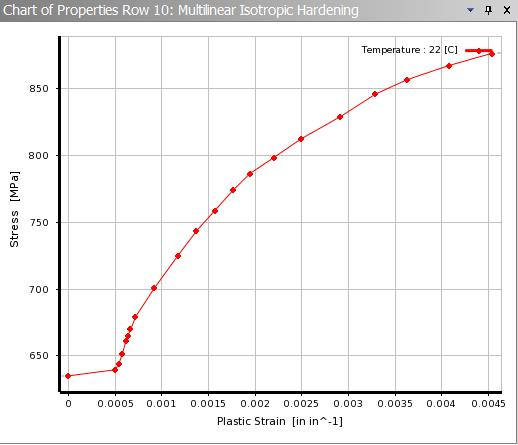In summary, the bolt stress after pretension is at 443/635 = 0.7 of yield strength.

The small fraction of an element in the bolt model that is over 635 MPa is shown below using a Capped Isosurface result display.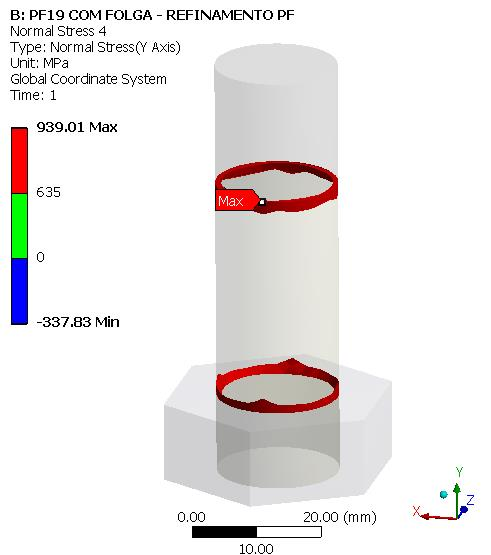It is only a fraction on an element that exceeds 635 MPa. Most of each element in the model is below yield.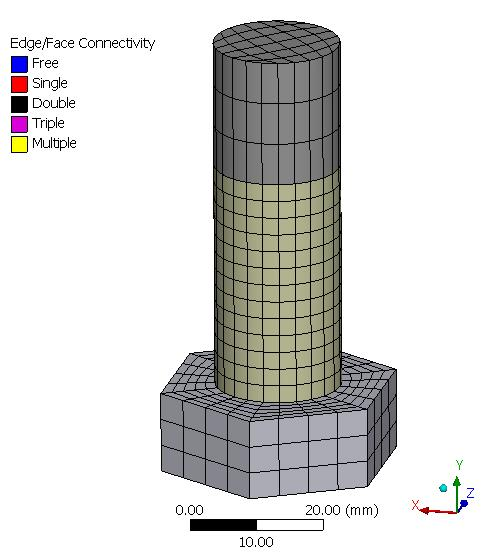I hope this clarifies the state of stress in the bolt at the end of the pretension step.

Regards,

Peter

• edited August 2018

Peter, split with solids it is not possible.

So Shall I slice the bolt in this plane that you showed, after that apply the pretension only in the face sliced, yes?If I understand it is what I should do.

• Fabricio,

I'm not sure what you are referring to, as there are two planes involved.

Plotting stress on a surface does not involve splitting any solids. The surface is just created to tell ANSYS where to plot the data.

When you use Bolt Pretension on the cylindrical face of a solid body, ANSYS automatically, and "under the hood", will split that solid body in order to apply the pretension load. You don't do the split manually, it does it for you.

You don't have to do anything unless you want to plot the stress on a surface, but you can get a similar result just using the section view too.

You can show your appreciation by clicking Like below the posts that are helpful.

• edited September 2018

Peter,

I think that there is problem with my bolts. Because when I take the stress results, with a 2x scale, I have the pictures below: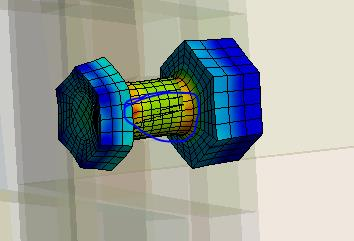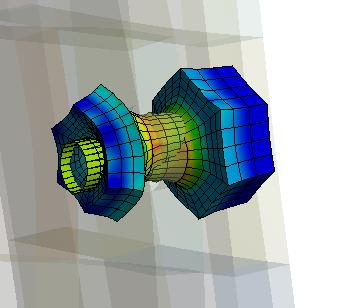I think that it occures because I applied the bolt pretension in the face of the thread. Is it correct?The shared topology is being used.

Thank you!!I saw it, because I need to show the deformation of the bolt in the enf of pretension load, and to see something I have to multiply the scale.

• edited October 2018

Hi Fabrico,

I think this is the same issue of Scale as discussed in another post.

Regards,

Ashish Khemka

• edited October 2018

Yes I Have asked for it in more than one post.